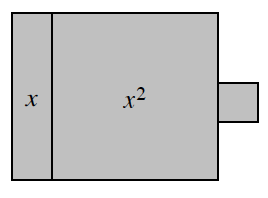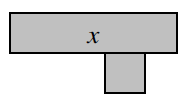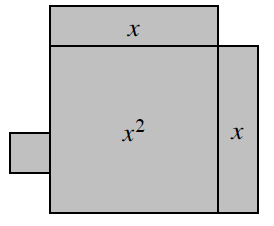### Home > CC2MN > Chapter 4 > Lesson 4.3.3 > Problem4-112

4-112.

Find the perimeter and area of each figure made of algebra tiles below.

1.The perimeter is the distance around a shape, so add the lengths of all the sides.
$x+1+x+x+1+1+x+1=4x+4$

To find the area, add the areas of the different parts of the figure, which are labeled in the problem.
$x^2+x+1$

Perimeter: $4x+4$ units
Area: $x^2+x+1$ units2

1.Follow the steps from part (a). It may help to label the sides of the figure.

The perimeter of the figure is $x+1+x+1+1+1=2x+4$ units.

1.Remember that the area of the figure is the sum of the labels of each tile, since the tiles are named for their area.